Refer to our Texas Go Math Grade 5 Answer Key Pdf to score good marks in the exams. Test yourself by practicing the problems from Texas Go Math Grade 5 Unit 6 Assessment Answer Key.

Vocabulary

Choose the best term from the box.

Question 1.
_____ is the income that is left after taxes. (p. 629)
Net income is the income that is left after taxes.

Question 2.
A ________ is money added to the cost of items or services. (p. 623)
A Sales Tax is money added to the cost of items or services.

Question 3.
________ is a portion of the value of an item, such as land. (p.623)
Property Tax is a portion of the value of an item, such as land.

Question 4.
The total income before any taxes are taken out is _____. (p. 629)
The total income before any taxes are taken out is Gross income.

Go Math Unit 6 Assessment Guide Grade 5 Pdf Question 5.
_____ is money that is paid to a City, state, or the U.S. government based on earnings. (p.617)
Income Tax is money that is paid to a City, state, or the U.S. government based on earnings.

Question 6.
The monthly income for a family is $2,000. The family’s monthly budget is shown. Describe two ways the family could adjust their budget to put$300 in a savings account each month. TEK 5.10.EQuestion 7.
What are some advantages and disadvantages of using a debit card over a credit card? TEKS 5.10.C
1. we don’t have to carry cash with us. Cash isn’t easy to track.
2. Debit cards are widely accepted around the world.
3. Multiple debit cards can be associated with one account.
4. A debit card is issued by a bank that allows a user to immediately use money from an account.
1. No credit is allowed A debit card is linked to our bank account. There is no possibility of making any transaction on credit.
2. There can be several fees associated with your debit card activities.
3. If we forget our PIN, then we might not have the option to complete a transaction.
4. Debit card rewards are often limited in size and availability.
2. EMI facility If we plan on making a large purchase and don’t want to sink our savings into it, we can choose to put it on our credit card.
1. The biggest con of a credit card is the minimum due amount that is displayed at the top of a bill statement.
2. High interest rate. If we do not clear our dues by our billing due date, the amount is carried forward and interest is charged on it.

Question 8.
A family’s monthly income is $2,100. Their monthly budget is shown. How can the family balance their budget if their rent increases by$250? TEK 5.10EA family’s monthly income is $2,100. Monthly expenses$900 + $75 +$600 + $300 +$200 = $2,075 If their rent increases by$250
1,150 + $75 +$575 + $150 +$150 = $2,100 The family balanced the budget by decreasing$25 in food, $150 in Entertainment and$50 in clothing.
Explanation:
A family’s monthly income is $2,100. Their monthly budget is shown. Their rent increases by$250. By adding all the expenses the sum is $2,075. The family balanced the budget by decreasing$25 in food, $150 in Entertainment and$50 in clothing.

Martin gets a check for $25 for his birthday on May 8th. The next day, he spends S16 on CDs and$2.50 on stamps. Complete the table to calculate the amount Martin has in available funds now. TEKS 5.10.D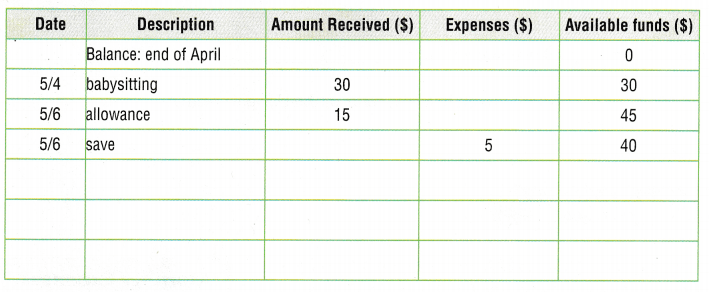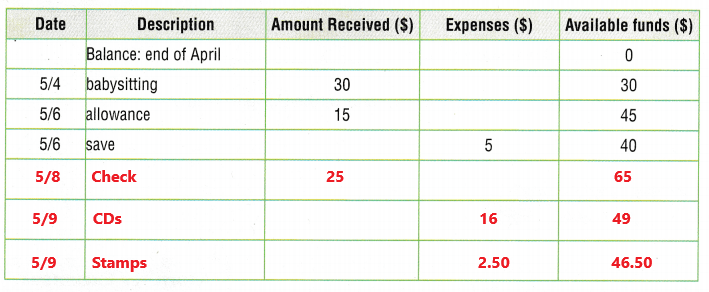The available funds on May 6 is $40. Martin gets a check for$25 for his birthday on May 8th.
$40 +$25 = $65 He spent$16 on CDs and $2.50 on stamps.$65 – $16 =$49
$49 –$2.50 = $46.50 Now Martin has the amount$46.50 in available funds.
Explanation:
In the above table we can observe amount received, expenses, available funds. The available funds on May 6 is $40. Martin gets a check for$25 for his birthday on May 8th. Add $40 with$25 the sum is $65. The next day, he spends S16 on CDs and$2.50 on stamps. Subtract $16 from$65 then the difference is $49. Subtract$2.50 from $49 the difference is$46.50. Now Martin has the amount $46.50 in available funds. Question 10. The total cost of an item is S98. If the item is purchased using automatic payments, the buyer will need to make four payments of$26. How much more does the item cost when using automatic payments? Explain. TEKS 5.10.C
The total cost of an item is $98. Automatic payments 4 x$26 = $104$104 – $98 =$6
The item cost is more $6 when using automatic payments. Explanation: The total cost of an item is S98. If the item is purchased using automatic payments, the buyer will need to make four payments of$26. Multiply $26 with 4 the product is$104. Subtract $98 from$104 the difference is $6. The item cost is more$6 when using automatic payments.

Question 11.
Sally got a pay raise. Her gross income increased by $500 and her net income increased by$417. By how much did Sally’s taxes increase? TEKS 5.10.B
$500 –$417 = $83 Sally’s taxes increases by$83.
Explanation:
Sally got a pay raise. Her gross income increased by $500 and her net income increased by$417. Subtract net income $417 from gross income$500 the difference is $83. So, Sally’s taxes increases by$83.

Texas Test Prep

Question 12.
Maura pays $0.02 in sales tax for every$1 that she spends. If Maura buys $60 worth of items, what is the total cost? TEKS 5.10.A (A)$60
(B) $0.02 (C)$1.20
(D) $61.20 Answer:$0.02 x $60 =$1.20
Sales tax on items is $1.20. The total cost is$60 + $1.20 =$61.20
So, option D is correct.
Explanation:
Maura pays $0.02 in sales tax for every$1 that she spends. Maura buys $60 worth of items. Multiply$0.02 with $60 the product is$1.20. The sales tax on items is $1.20. The total cost is calculated by adding$60 with $1.20 then the sum is$61.20. So, option D is correct.

Raul pays property tax in Dallas. On which of the following might Raul pay property tax? TEKS 5.10.A
Raul might pay property Taxes such as cars, houses, boats, or land.
Explanation:
Raul pays property tax in Dallas. Property tax is a portion of the value of items that is paid to a city or state government. Property tax can be charged on things such as cars, houses, boats, or land.

Question 14.
Lorena makes a list of the taxes she pays each month. How much does she pay in payroll tax? TEKS 5.10.A
(A) $418.24 (B)$21.35
(C) $177.59 (D)$240.65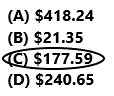$116.24 +$61.35 = $418.24 Lorena pays 177.59 in payroll tax. So, option C is correct. Explanation: Lorena makes a list of the taxes she pays each month. Add federal income tax and other payroll taxes because sales tax and property tax doesn’t come under payroll tax. Add$116.24 with $61.35 the sum is$177.59. Lorena pays 177.59 in payroll tax. So option C is correct.

Question 15.
Ralph makes a monthly budget. His monthly allowance is $40. He makes$25 each month from mowing the neighbor’s lawn. Ralph’s monthly expenses are $75. How much does he need to earn from babysitting to balance his budget? TEKS 5.10.F (A)$75
(B) $10 (C)$50
(D) $35 Answer:Ralph’s income is$40 + $25 =$65
Ralph’s monthly expenses are $75.$75 – $65 =$10
To balance his budget Ralph need to earn $10 from babysitting. So, option B is correct. Explanation: Ralph makes a monthly budget. His monthly allowance is$40. He makes $25 each month from mowing the neighbor’s lawn. Add$40 to $25 the sum is$65. Ralph’s monthly expenses are $75. Subtract income$65 from expenses $75 then the difference is$10. To balance his budget Ralph needs to earn $10 from babysitting. So, option B is correct. Go Math 5th Grade Unit 6 Assessment Answer Key Question 16. Which of the following can Chaseedah use to purchase an item if she wants to pay for her purchase at a later time? TEKS 5.10.C (A) debit card (B) check (C) money transfer (D) credit card Answer: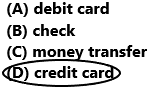Option D is correct. Explanation: Chaseedah can use to purchase an item and she can pay for her purchase at a later time by using credit card. So option D is correct. Fill in the bubble completely to show your answer. Question 17. The price tags at a clothing store do not include the sales tax in the prices. Chase buys$210 worth of clothing at the store. He hands the cashier $250 and receives three$5 bills and 89 cents in change. How much sales tax does Chase pay on his purchase? TEKS 5.10.A
$250 –$210 = $40 Chase has to receive$40 in return.
He receives three $5 bills and 89 cents in change. 3 x$5 + 0.89 = $15.89 He receives$15.89.
$40 –$15.89 = $24.11 Chase pay$24.11 as sales tax on his purchase.
Explanation:
The price tags at a clothing store do not include the sales tax in the prices. Chase buys $210 worth of clothing at the store. He hands the cashier$250 and receives three $5 bills and 89 cents in change. First subtract$210 from $25o the difference is$40. Chase has to receive $40 in return. But he receives three$5 bills and 89 cents in change. He receives $15.89. Subtract$15.89 from $40 the difference is$24.11. Chase pay $24.11 as sales tax on his purchase. Question 18. Reema pays$11.05 in property tax for every $1,000 in value of her land. If Reema’s land is worth$100,000, what will she pay in property tax? TEKS 5.10.A
(A) $1,000 (B)$11.05
(C) $100,ooo (D)$1,105$1,000 =$11.05
Reema’s land is worth $100,000$100 x $1,000$100 x $11.05 =$1,105
Reema pays $1,105 in property tax. So, option D is correct. Explanation: Reema pays$11.05 in property tax for every $1,000 in value of her land. If Reema’s land is worth$100,000. Multiply $100 with$11.05 the product is $1,105. Reema pays$1,105 in property tax. So, option D is correct.

Go Math Grade 5 Answer Key Unit 6 Formative Assessment Question 19.
The Perry family’s monthly net income is $2,900. They budgeted$1,200 for rent, $600 for food,$600 for clothing, $500 for entertainment, and$200 for travel expenses. Which of the following actions
results in a balanced budget? TEK 5.10E
(A) Increase spending on food by $100. (B) Decrease spending on food by$20.
(C) Decrease spending on entertainment by $200. (D) Increase spending on entertainment by$200.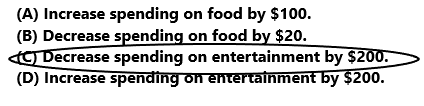Perry’s income is $2,900. Perry’s expenses$1,200 + $600 +$600 + $500 +$200 = $3,100 To get the balanced budget Perry needs to decrease spending on entertainment by$200.
So, option C is correct.
Explanation:
The Perry family’s monthly net income is $2,900. They budgeted$1,200 for rent, $600 for food,$600 for clothing, $500 for entertainment, and$200 for travel expenses. Add all the expenses the sum is $3,100. To get the balanced budget Perry needs to decrease spending on entertainment by$200. So, option C is correct.

5th Grade Go Math Unit 6 End of Assessment Answer Key Question 20.
Gina’s gross monthly income is $1,000. The pay stub shows the amount of taxes she pays each month. What is Gina’s net monthly income? TEKS 5.10.B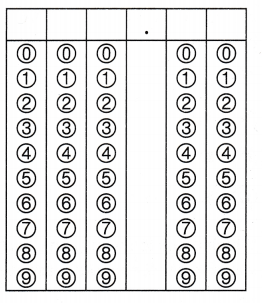Record your answer and fill in the bubbles on the grid. Be sure to use the correct place value.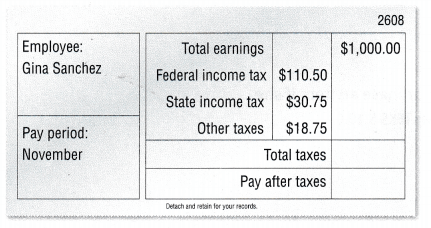Answer: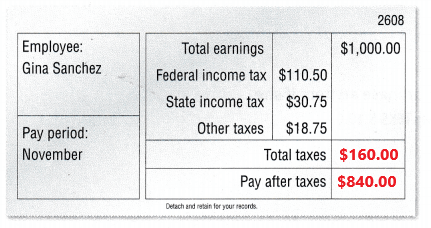Gina’s gross monthly income is$1,000.
Total taxes
$110.50 +30.75 + 18.75 =$160.00
Net monthly income
$1,000 –$160.00 = $840.00 Gina’s net monthly income is$840.00.
Explanation:
Gina’s gross monthly income is $1,000. The pay stub shows the amount of taxes she pays each month. Add all the taxes. Add$110.50 with $30.75 and$18.75 the sum is $160.00. Gina’s total taxes are$160.00. Subtract $160.00 from$1,000 the difference is $840.00. Gina’s net monthly income is$840.00.

Scroll to Top